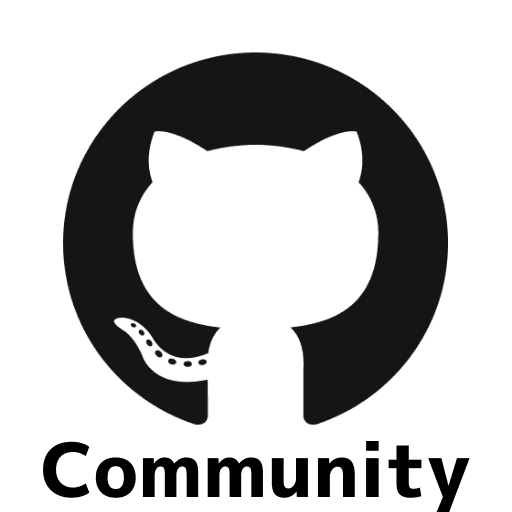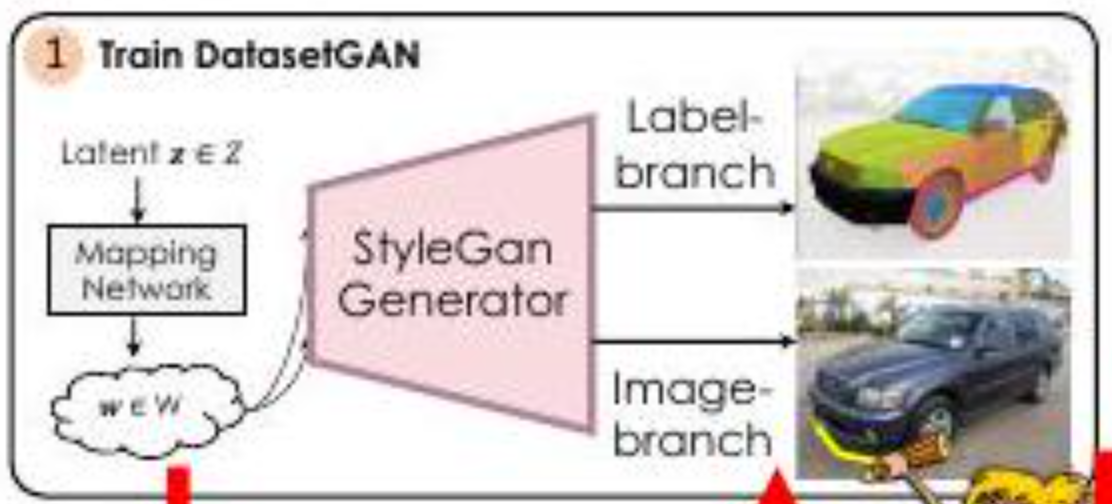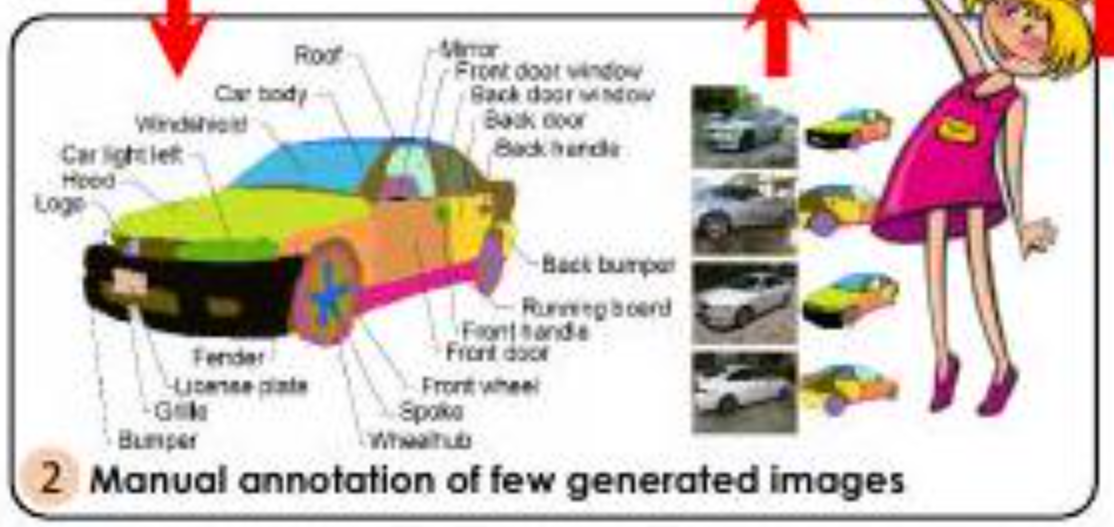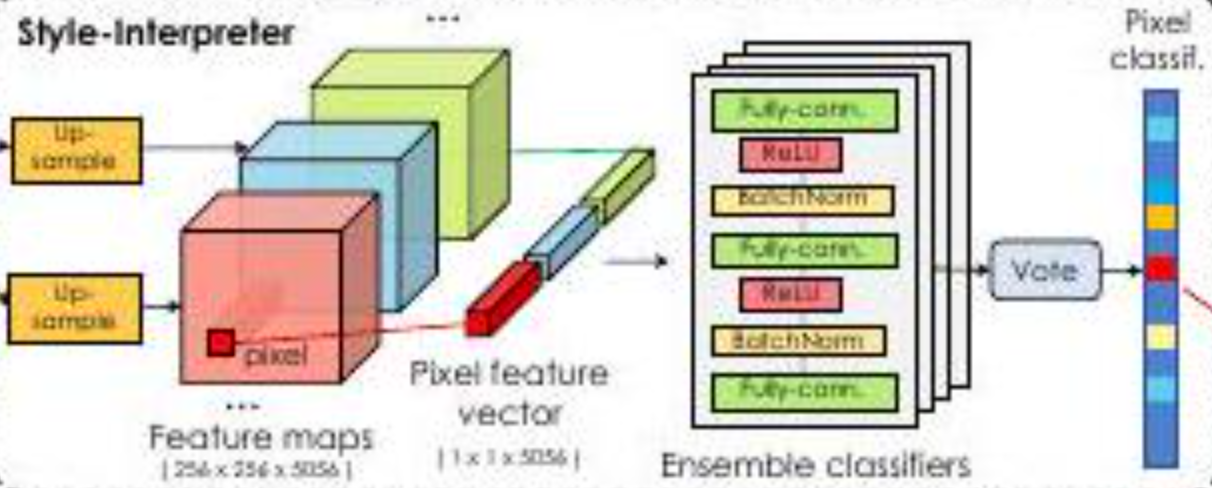# Catch up on the latest AI articles# A GAN-based Image Generation Method That Revolutionizes The Generation Of Annotated Datasets

3 main points
✔️ DatasetGAN is a tool for generating detailed annotated images.
✔️ A human only needs to assign detailed annotations to a very small amount of images (16 images), which can generate an infinite number of annotated datasets.
✔️ Semi-supervised learning was performed on the generated images and achieved performance comparable to fully supervised learning.

written by Yuxuan ZhangHuan LingJun GaoKangxue YinJean-Francois LaflecheAdela BarriusoAntonio TorralbaSanja Fidler
(Submitted on 13 Apr 2021 (v1), last revised 20 Apr 2021 (this version, v2))
Comments: Accepted to CVPR 2021 as an Oral paper

Subjects: Computer Vision and Pattern Recognition (cs.CV)code：The images used in this article are from the paper, the introductory slides, or were created based on them.

## Introduction.The core of this paper is presented in the introduction. As shown in the figure above, StyleGAN generates segmented images and normal images (cars). This allows us to generate an unlimited number of annotated images and use those datasets for classification, identification, etc." is the claim of this paper.Furthermore, only a small amount of manual annotation is required. In the paper, only 16 images were annotated, and the annotated images were successfully generated.

Additionally, the annotations are detailed as shown in the figure above. Annotations are assigned to each part of the car (front lights, pumper, grille, etc.). Assigning such detailed (high-quality) annotations takes time and manpower (i.e., money). The benefits of saving time, manpower, and money are reiterated in this paper.

## General solution for no or few annotations

### How to use data synthesis

There are known studies that use 3DCG to create annotated images (datasets) and studies that generate datasets with GAN (Generative Adversarial Network). This study also uses GAN, but prior studies assumed a large labeled dataset.

### semi-supervised learning

When using a segmentation network, this network can be thought of as a generator of annotations. This network learns better segmentations with a small amount of supervision (manual annotation). This can be used on unannotated images to obtain pseudo-labels (pseudo-segmentation).

It is similar to this paper in that it generates a segmentation image, but the major difference is the source image. While the previous study requires an unlabeled source image, this study generates even that image.

### Contrastive learning

Contrast learning is an unsupervised learning method that learns whether a given pair of images is similar. For example, one image is divided into two parts, which are then considered as a pair. Since the original images are identical, the pair is considered to be in the same class. Conversely, a pair consisting of two randomly selected images is considered to be in a different class. In contrast learning, image features are acquired by learning whether the paired images are of the same class.

Contrast learning can be applied to the segmentation task by further fine-tuning (fine-tuning) the image segmentation (patches) and by fine-tuning with annotated images.

## Proposed Methodology for this Study

### What is StyleGAN?In this paper, StyleGAN is used to generate images and their segmentation images (= annotated images). For the sake of understanding, we first explain StyleGAN.

A GAN performs a specific task by having two models compete with each other (in this case, image generation): the one that generates the image (the generator) and the one that detects whether the image is generated (the discriminator). By having these two compete, the generator will produce a more realistic image, one that cannot be detected as being a generated image.

The generator is fed a vector (latent variable) consisting of random numbers created from a normal distribution. In this paper, it is written as $z \in Z$. This vector is repeatedly transformed, and the end result is a tensor representing the image data.

The latent variable $z$ is transformed by being input into a network (CNN or MLP) called a mapping function. This transformed vector is called the intermediate latent variable $w$. More precisely, the input to the generator is $w$, and the subsequent upsampling process is called the generator.

The vector (tensor) upsampled to the desired size is denoted by $S$.

So far we have outlined the generators; in StyleGAN there are $k$ more ways to convert $z$ to $w$. The resulting $k$ vectors $w_{1}, w_{2}, w_{3}, \dots, w_{k}$ are called Style. Thus, from $k$ styles, $k$ upsampled tensors $S_{1}, S_{2}, S_{3}, \dots, S_{k}$ are generated.

### Key Points of this MethodThe paper states that "latent variables are regarded as graphics and StyleGAN as a rendering engine. In other words, the authors believe that latent variables define "what kind of object is there" and StyleGAN defines "how it looks like. Or perhaps it is easier to simply think of the latent variable as the subject and StyleGAN as the camera.

Therefore, the authors believe that the resulting $S_{1}, S_{2}, S_{3}, \dots, S_{k}$ have (should have) dimensions that control the visibility of objects.

Then $S_{1}, S_{2}, S_{3}, \dots, S_{k}$ are concatenated in the depth direction to obtain a 3-dimensional tensor (the "feature maps" part of the figure). Then, focusing on a specific pixel, a vector in the depth direction is obtained. This is called the pixel feature vector and is represented as $S_{i,*}$. In other words, the part corresponding to each pixel (= pixel feature vector) is extracted in advance from the feature map that will become an image in the future.

The pixel feature vectors are then input to the MLP, which consists of three layers. It then learns which pixel feature vector corresponds to which pixel by comparing it to the actual generated image. In this way, it learns which pixels correspond to which class and generates a segmentation image for the image as a whole.

### Loss function in image generation

Segmentation failures are especially visible as noise (pixels of one class are mixed with pixels of another class, and no partition is created that consists of a specific class).

To evaluate this labeling failure, the Jensen-Shannon (JS) divergence (hereafter $D_{JS}$) is introduced.

$$D_{JS}(P \| Q)=\alpha D_{K L}(P \| M)+\alpha D_{K L}(Q \| M),$$

$$M = \alpha(P+Q),$$

$$D_{KL}(P \| Q)=-\sum_{x \in X} P(x)\log \frac{Q(x)}{P(x)},$$

$$P_{(x)}=p(X=x),$$

$$Q_{(x)}=p(X=x \mid a)$$

$D_{KL}$ is the Kullback-Leibler divergence (KL information measure), which evaluates the similarity of probability distributions. $D_{JS}$ is an improved version of this. In this paper, the JS divergence is used to evaluate the uncertainty of the synthesized images.Above is an example of an image synthesized by DATASETGAN. You can see the high quality of the composite image and its segmentation (although, in fact, there are no wrinkles on the forehead of the person and unsegmented parts of the cat's body. (The teacher data is not like that).

### Comparison with human annotation

In this case, we compared the results with one experienced annotator (using LabelMe). The generated images include a bedroom, a car, a human head (face), a bird, and a cat.Partonomy is a tree structure that shows the relationship between parts of an object. For example, in the case of a human face, the "nose" is a branch of the "head," and the "nose tip" and "nostrils" are derived from that branch. The figure above is an example of visualizing partonomy.

Partnomies were defined manually for each class and were as detailed as possible. In the case of heads, an average of 58 parts were annotated. To annotate these manually would have taken about 20 minutes per piece. However, with our DatasetGAN method, it takes only about 9 seconds per piece.

Manually annotating 10,000 images takes about 4 months (134 days), or more than a year if you annotate 8 hours a day. With DatasetGAN, this takes only 25 hours.

Even more surprisingly, the training of the Style-Interpretor was actually completed with only 16 images of heads, 16 images of cars, 30 images of birds, 30 images of cats, and 40 images of bedrooms. If we limit ourselves to annotating only faces, we were able to generate an infinite data set in about 5 hours of annotation work (conversely, that's how good the StyleGAN trained model on which it is based is).These are generated images of birds, cats, and bedrooms. Although the number of classes is smaller than that of the human head, the annotation itself was more difficult and the time taken was longer than that of the head.

## Evaluation of generated images

### Model used

Segmentation was performed on a model called Deeplab-V3, which is a network for segmentation with ResNet151 as its backbone. is trained on DatasetGAN generated images.

### Models for comparison

Comparison of Transition Learning

For comparison, we use Deeplab-V3, which has already been trained with MS-COCO. With this model, only the final layer is tuned (fine-tuning) with data from faces, cats, birds, etc. The annotation of the training data in this case is done by human.

Comparison of semi-supervised learning

For semi-supervised learning, the model in Reference  (Semi-Supervised Semantic Segmentation with High- and Low-level Consistency ) is used for comparison. Reference  also reports on a segmentation task using GANs and proposes a method to reduce segmentation noise by using two GANs.

This model uses the pre-trained weights from ImageNet. The model is trained using human-annotated teacher data and the same unannotated data as trained by StyleGAN (not images synthesized by DatasetGAN, but images used for pre-training by the Style-Interpretor within DatasetGAN). (These are not images synthesized by DatasetGAN.)

Fully supervised learning

Fully supervised training of Deeplab-V3 on several datasets.

## resultThe graph above shows the results using a dataset of car images called ADE-Car12. The horizontal axis is the number of images used for training (in thousands) and the vertical axis is the average IOU.

The red dotted line is fully supervised learning and the blue is the proposed method in this paper. Green is semi-supervised learning and orange is transition learning, but they perform better than the proposed method than the compared semi-supervised learning and transition learning.

Even more interesting is the fact that the performance is equivalent to fully supervised learning when training with more than 20,000 cards.This table shows the results for different datasets. In any case, we can see that the proposed method outperforms the other methods.

## Conclusion.

This paper introduced DatasetGAN for dataset generation.

DasetGAN has shown that an effective segmentation task can be learned from a few manual annotations by using StyleGAN's feature maps. This allows for the synthesis of large annotated datasets.

Training with images synthesized with DatasetGAN was shown to perform better than general transition learning and semi-supervised learning models that do not use synthesized images. In addition, several test datasets showed that when the number of training data is increased with DatasetGAN, the performance is comparable to fully supervised learning.

If you have any suggestions for improvement of the content of the article,